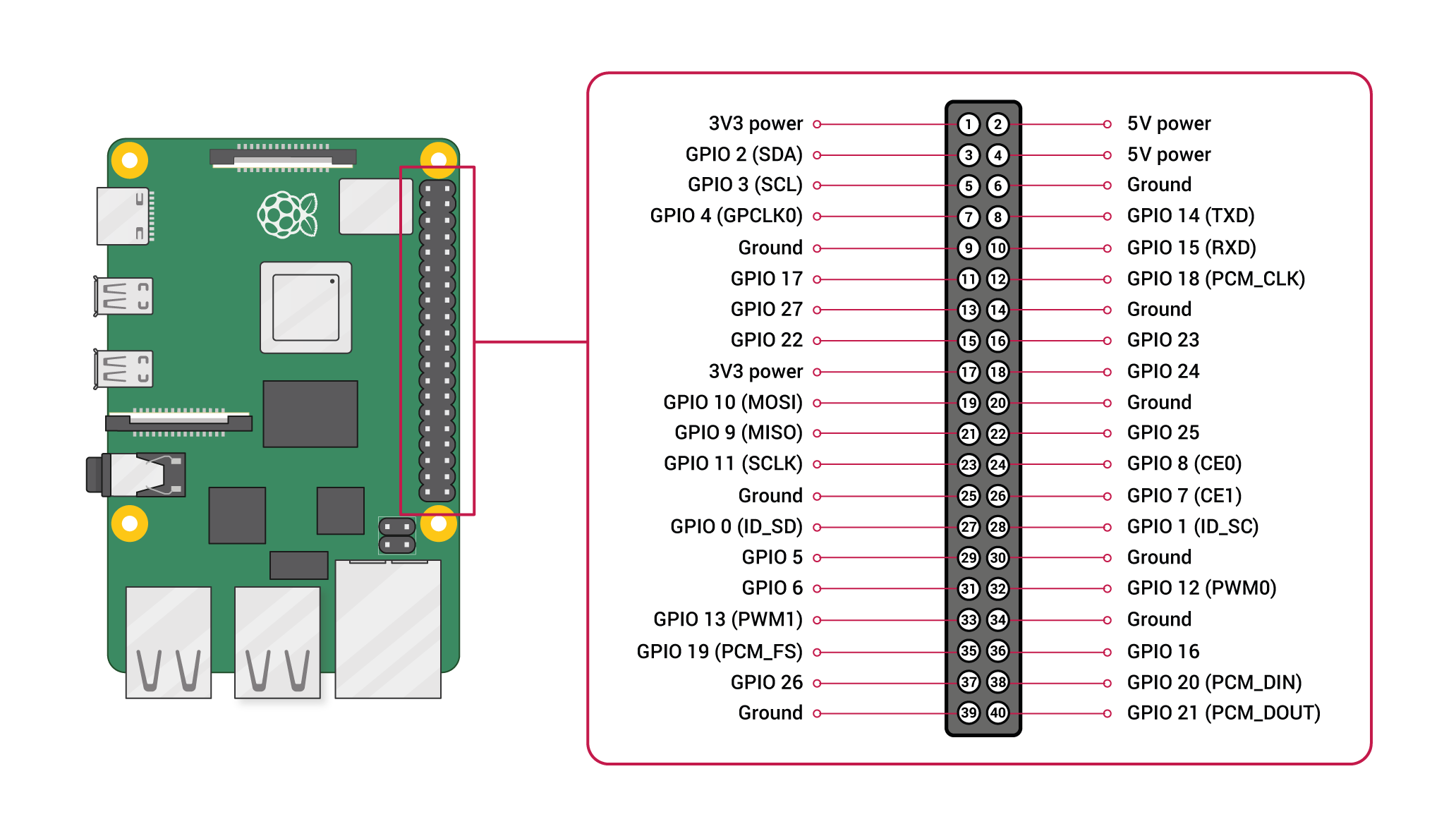Notesclub

# Pulse Width Modulation Using Pigpiox

## Prerequisites

• Raspberry Pi - This example uses `pigpiox` which won’t work on other boards
• LED
• 220 Ohm resister

## Introduction

In this exercise, we will leverage the pigpiox library to brighten and dim an LED through software PWM (pulse width modulation).

## Try it out

Let’s set a variable so that we don’t have to choose the GPIO pin every time.

First, refer to the GPIO ports for your Nerves device. In this example, we will be using pin 20 on the Raspberry Pi platform.``led_pin = 20``

Alright! Now that we’ve selected a pin, make sure that we connect the to our `led_pin` and `ground` through a 220Ω resistor. The long leg can be connected to our `led_pin` and the short pin can connect from the shorter leg to a 220Ω resistor, then to our ground.Now we will create a connection to that GPIO pin and set it as an `:output`.

``Pigpiox.GPIO.set_mode(led_pin, :output)``

After our `led_pin` has been configured as an `:output`, let’s turn it on at 10% brightness.

``````#Pigpiox.Pwm.gpio_pwm(led_pin, 255) # 100%
#Pigpiox.Pwm.gpio_pwm(led_pin, 127) # 50%
Pigpiox.Pwm.gpio_pwm(led_pin, 25)  # 10%
#Pigpiox.Pwm.gpio_pwm(led_pin, 2)   # 1%``````

As you can see above, you can run the PWM cycles between `0` and `255`, or the max size of a byte.

Let’s go ahead and write a basic `defmodule` that can dim or brighten the LED using recursion.

``````defmodule PWM do
# Repeat brightening forever
def forever(output_gpio, brightness \\ 0, delay \\ 10, direction \\ true) do
Pigpiox.Pwm.gpio_pwm(output_gpio, brightness)
Process.sleep(delay)
# Increase the brightness by 1 ever iteration until max, then return to zero.
brightness = if direction, do: brightness + 1, else: brightness - 1
# flip the direction if over max u8 or at min u8
direction = if brightness > 254 or brightness < 1, do: !direction, else: direction
# Recursively call the function
forever(output_gpio, brightness, delay, direction)
end
end

PWM.forever(led_pin)``````

For a fun exercise, see if you can change the `PWM.forever` function to only brighten to 50% in the forever loop.